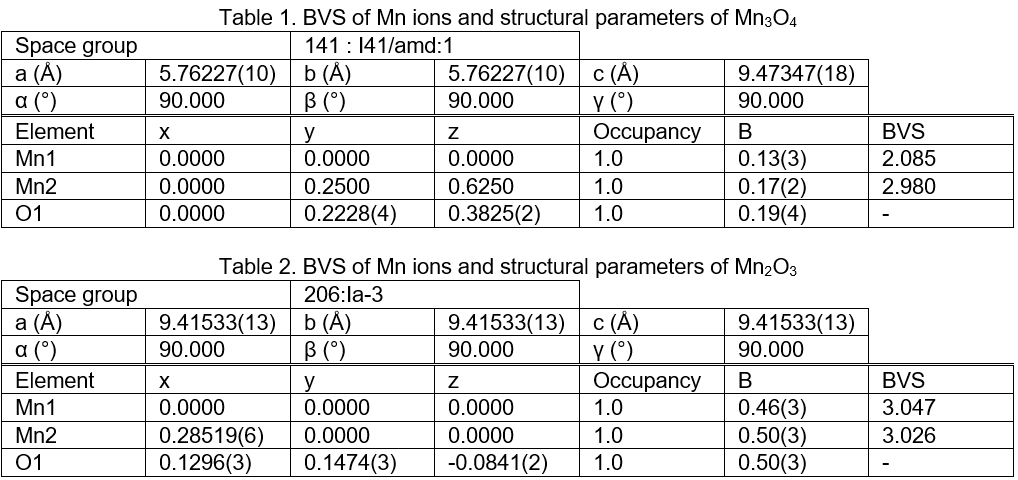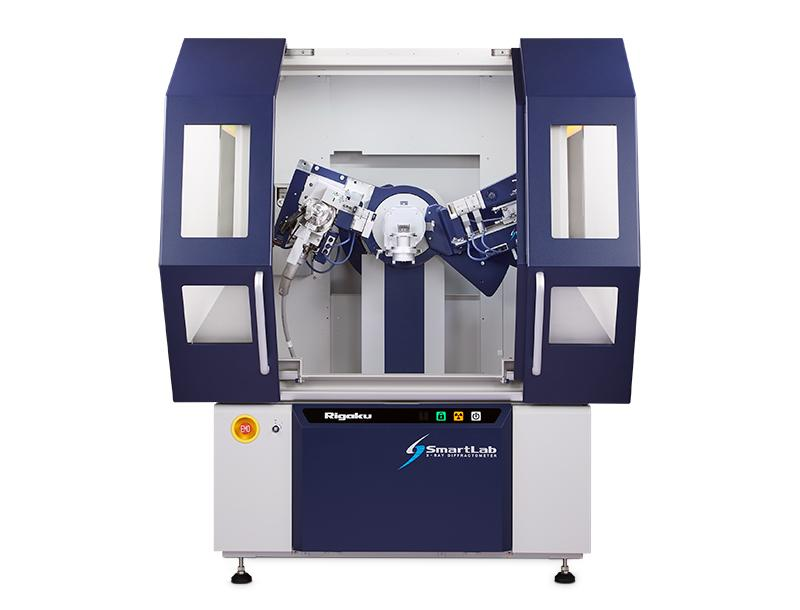# EVALUATION OF OXIDATION STATE BY THE BVS METHOD

### Introduction

The valence of metal ions in a crystal is an important structural parameter for understanding a material’s property and for designing new materials. There are numerous methods of experimentally determining the valence of a metal ion, including XAFS (X-ray Absorption Fine Structure), XPS (X-ray Photoelectron Spectroscopy), Mössbauer absorption spectroscopy, MEM (Maximum Entropy Method) and BVS (Bond Valence Sum). BVS is an empirical method based on Rietveld analysis that estimates the valence states based on the bond distances to neighboring atoms. Here, we illustrate the oxidation states of Mn oxides by BVS.

Measurements and Results

X-ray powder diffraction of Mn3O4 and Mn2O3 were performed using Cu radiation. X-ray fluorescence of Mn is removed by the XRF reduction mode of the detector. The d -spacing is measured to be 0.82 Å (2θ/θ = 140˚), which corresponds to half to a third of the length of the Mn-O bond distance. BVS, which is computed from the Mn-O bond distances obtained from the Rietveld analysis, and the Bond Valence Parameters (Mn2+-O2-:1.790 (3) and Mn3+-O2-:1.760 (5)) (1), predicts the proper oxidation state of the Mn ion for a given crystal structure as shown in Fig.1. Table 1 and 2 show the BVS values for Mn ions in the Mn3O4 and Mn2O3 crystal structures, respectively. The reliability factors of the Rietveld analysis are Rwp = 4.49%、Rp = 2.62%、S = 1.6093 for Mn3O4, and Rwp = 4.55%, Rp = 3.59%, S = 1.1949 for Mn2O3, respectively. BVS is useful for evaluating the stability and the validity of artificially designed crystal structures, in addition to being a simple approach for predicting and estimating the oxidation states of metal ions from the crystal structure.Fig 1. The input screen for the BVS calculation of Mn3O4. (When the Mn valences are input as trivalent, the BVS of Mn1 and Mn2 sites are calculated as 1.92 (divalent) and 2.98 (trivalent), respectively.)References: (1) I. D. Brown and D Altermatt: Acta Cryst., B41 (1985) 244-247.
Recommended equipment and software
► Automated multipurpose X-ray diffractometer SmartLab (SmartLab SE)
► Integrated X-ray analysis software SmartLab Studio II (Powder XRD plugin)## SmartLab